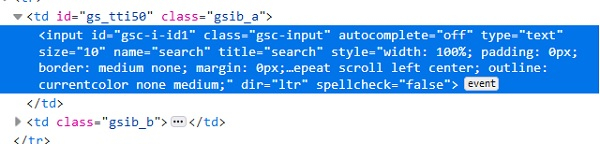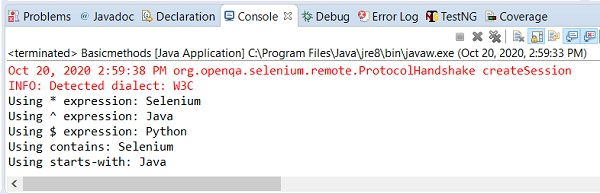# How to use regex in selenium locators?

We can use regex in locators in Selenium webdriver. This can be achieved while we identify elements with the help of xpath or css locator. Let us have a look at the class of an element in its html code. The class attribute value is gsc-input.Here, with the css expression, we can use the * and perform a partial match with the class attribute value. The css value shall be input[class*='input']. This means the subtext input is present in the actual text gsc-input. We can also use the ^ and perform a match with the class. The css value shall be input[class^='gsc']. This means the actual text gsc-input starts with the subtext gsc. We can also use the $and perform a match with the class. The css value shall be input[class$='put']. This means the actual text gsc-input ends with the subtext put.

Here, with the xpath expression, we can use the contains() method and perform a partial match with the class. The xpath value shall be //*[contains(@class, 'input')]. This means the subtext input is present in the actual text gsc-input. We can also use the starts-with() and perform a match with the class. The css value shall be //*[starts-with(@class, 'gsc')]. This means the actual text gsc-input starts with the subtext gsc. We can also use the ends-with() and perform a match with the class. The css value shall be //*[ends-with(@class, 'put')]. This means the actual text gsc-input ends with the subtext put.

## Example

Code Implementation.

import org.openqa.selenium.WebDriver;
import org.openqa.selenium.chrome.ChromeDriver;
import org.openqa.selenium.WebElement;
import org.openqa.selenium.By;
public class RegexLocator{
public static void main(String[] args) {
System.setProperty("webdriver.chrome.driver", "C:\Users\ghs6kor\Desktop\Java\chromedriver.exe");
WebDriver driver = new ChromeDriver();
// identify element with partial class match with * in css
WebElement l=driver.findElement(By.cssSelector("input[class*='input']"));
l.sendKeys("Selenium");
// obtain the value entered with getAttribute method
System.out.println("Using * expression: " +l.getAttribute("value"));
l.clear();
// identify element with partial class match with ^ in css
WebElement m=driver.findElement(By.cssSelector("input[class^='gsc']"));
m.sendKeys("Java");
// obtain the value entered with getAttribute method
System.out.println("Using ^ expression: " +m.getAttribute("value"));
m.clear();
// identify element with partial class match with $in css WebElement n = driver.findElement(By.cssSelector("input[class$='put']"));
n.sendKeys("Python");
// obtain the value entered with getAttribute method
System.out.println("Using \$ expression: " +n.getAttribute("value"));
n.clear();
// identify element with partial class match with contains in xpath
WebElement o= driver.findElement(By.xpath("//input[contains(@class,'input')]"));
o.sendKeys("Selenium");
// obtain the value entered with getAttribute method
System.out.println("Using contains: " +o.getAttribute("value"));
o.clear();
// identify element with partial class match with starts-with in xpath
WebElement p= driver.findElement(By.xpath("//input[starts-with(@class,'gsc')]"));
p.sendKeys("Java");
// obtain the value entered with getAttribute method
System.out.println("Using starts-with: " +p.getAttribute("value"));
p.clear();
driver.close();
}
}

## Output